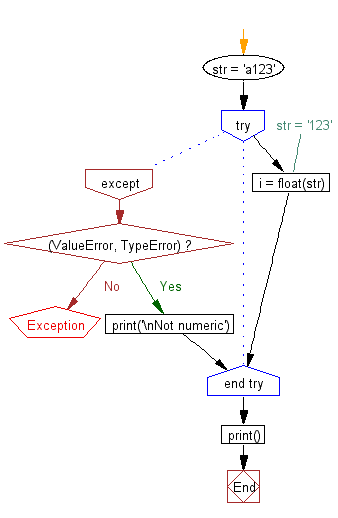﻿ Python: Check whether a string is numeric - w3resource# Python: Check whether a string is numeric

## Python Basic: Exercise-95 with Solution

Write a Python program to check whether a string is numeric.

Sample Solution-1:

Python Code:

``````str = 'a123'
#str = '123'
try:
i = float(str)
except (ValueError, TypeError):
print('\nNot numeric')
print()
```
```

Sample Output:

```Not numeric
```

Flowchart:Sample Solution-2:

Python Code:

``````# Doesn't work for floats
text = input("Input a word or numbers: ")
if text.isdigit():
print("The input value is numbers.")
else:
print("The input value is string.")
```
```

Sample Output:

```Input a word or numbers:  a123
The input value is string.
```

Python Code Editor:

Have another way to solve this solution? Contribute your code (and comments) through Disqus.

What is the difficulty level of this exercise?

Test your Python skills with w3resource's quiz

﻿

## Python: Tips of the Day

Try-catch-else construct:

```try:
foo()
except Exception:
print("Exception occured")
else:
print("Exception didnt occur")
finally:
print("Always gets here")
```